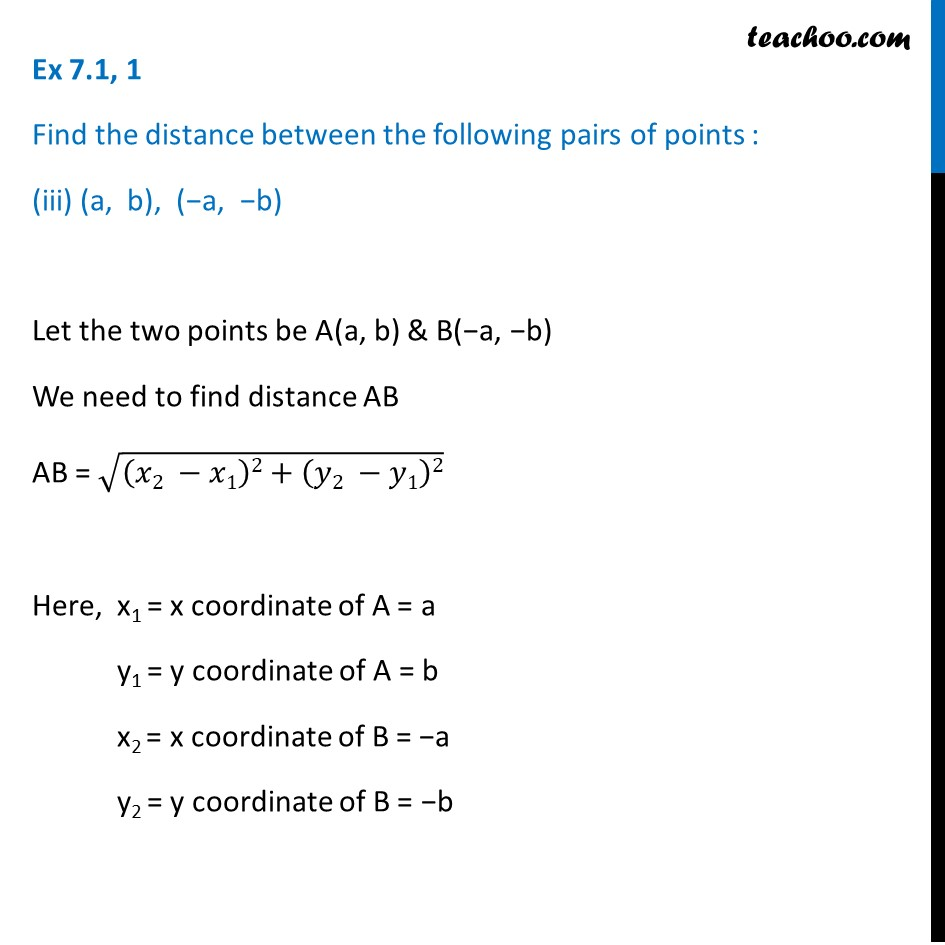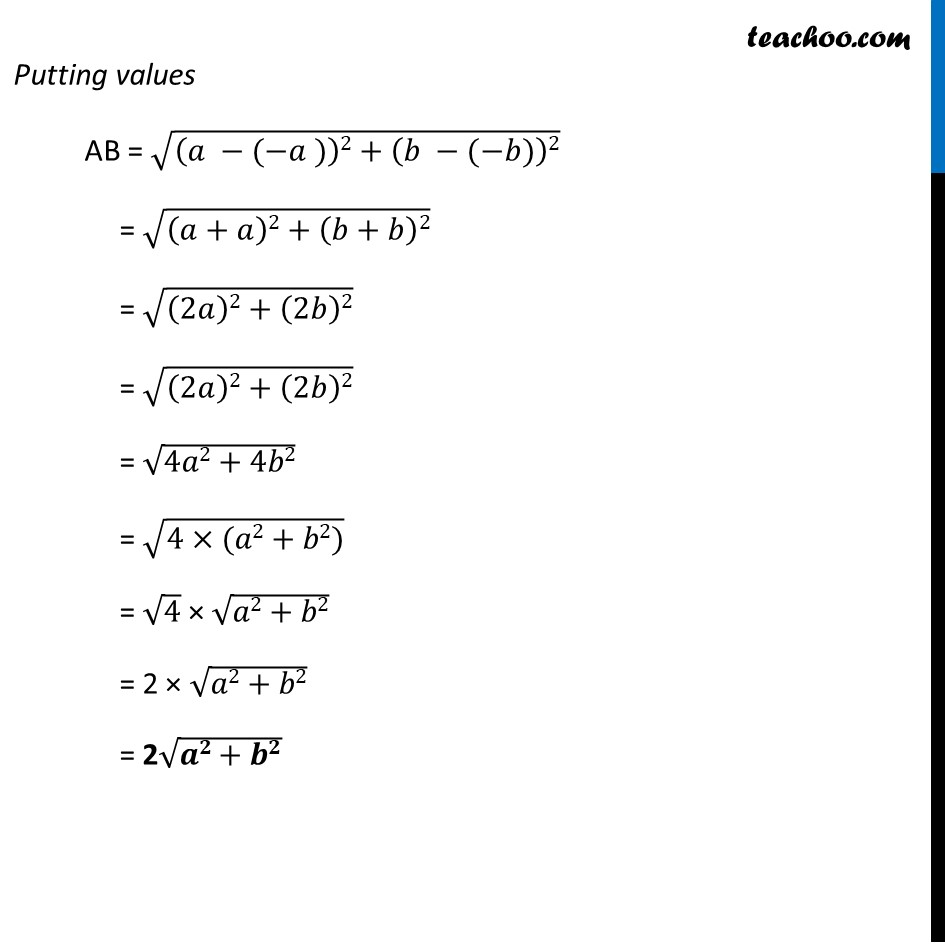Ex 7.1

Chapter 7 Class 10 Coordinate Geometry
Serial order wiseLearn in your speed, with individual attention - Teachoo Maths 1-on-1 Class

### Transcript

Ex 7.1, 1 Find the distance between the following pairs of points : (a, b), (−a, −b) Let the two points be A(a, b) & B(−a, −b) We need to find distance AB AB = √((𝑥2 −𝑥1)2+(𝑦2 −𝑦1)2) Here, x1 = x coordinate of A = a y1 = y coordinate of A = b x2 = x coordinate of B = −a y2 = y coordinate of B = −b Putting values AB = √((𝑎 −(−𝑎 ))2+(𝑏 −(−𝑏))2) = √((𝑎+𝑎)2+(𝑏+𝑏)2) = √((2𝑎)2+(2𝑏)2) = √((2𝑎)2+(2𝑏)2) = √(4𝑎2+4𝑏2) = √(4×(𝑎2+𝑏2)) = √4 × √(𝑎2+𝑏2) = 2 × √(𝑎2+𝑏2) = 2√(𝒂𝟐+𝒃𝟐)